### Home > A2C > Chapter 10 > Lesson 10.1.2 > Problem10-53

10-53.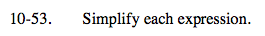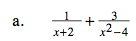$\frac{1}{x+2}+\frac{3}{(x+2)(x-2)}$

$\frac{(x-2)}{(x+2)(x-2)}+\frac{3}{(x+2)(x-2)}$

$\frac{x+1}{x^{2}-4}$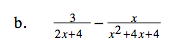See part (a).

$\frac{x+6}{2(x+2)^{2}}$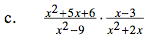Factor before trying to simplify.

$\frac{1}{x}$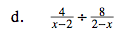$\frac{4}{x-2}\div \frac{8}{-(x-2)}$